# Summing Stock Lists

In order to calculate sum of stock lists we will multiply the quantity with price of stock, we will use SUM & SUMPRODUCT function to get the output.

SUM: Adds all the numbers in a range of cells

Syntax: =SUM(number1,number2,...)

There can be a maximum of 255 arguments refer below screenshot: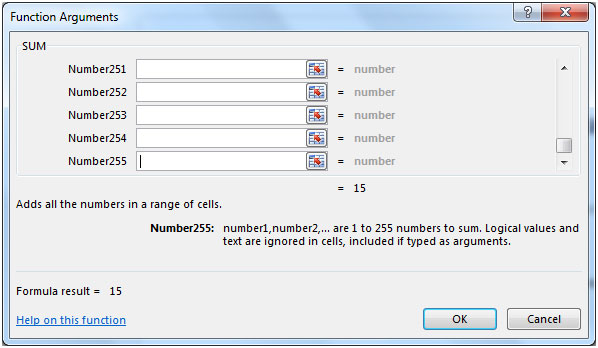SUMPRODUCT: Returns the sum of the products of corresponding ranges or arrays.

Syntax: =SUMPRODUCT(array1,array2,array3,...)

There can be maximum of 255 arrays in Sumproduct function refer below screenshotLet us take an example:

In order to calculate the Price after multiplying each cell value from Stock List with the corresponding Qty&then take sum of all the prices, we can use SUM & SUMPRODUCT functions.

1. Price = Stock List x Qty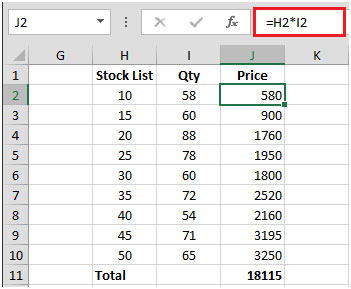2. Copy the formula to below range of cells & then take total of all the Price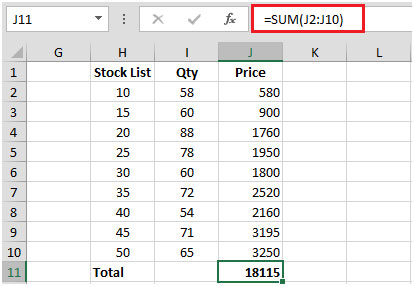Column A contains list of stock. Column B contains quantity. Column C contains stock price. In order to calculate the price we need to calculate the sum by multiplying quantity & price & then take sum.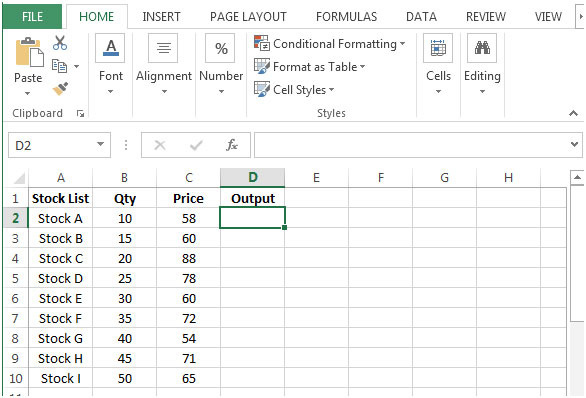• In D2 the formula would be
• {=SUM((\$B\$2:\$B\$10)*(\$C\$2:\$C\$10))}
• This is an array formula which requires formula to be enclosed with curly brackets by using CTRL + SHIFT + ENTER.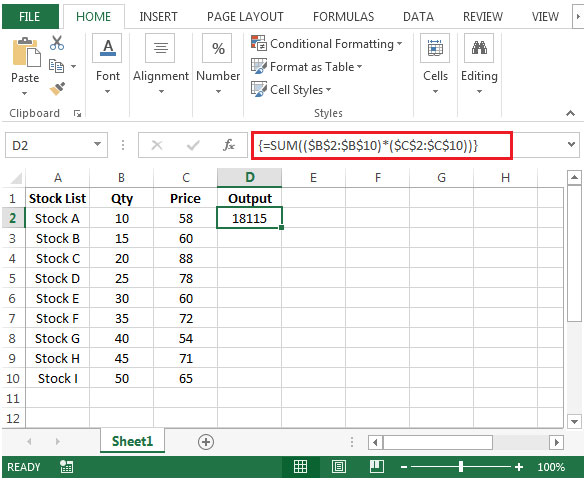There is another way by which we can find the same result by using SUMPRODUCT function.

• In cell E2 the formula would be
• =SUMPRODUCT((\$B\$2:\$B\$10)*(\$C\$2:\$C\$10))
• Press Enter on your keyboard.
• The function will return the same result.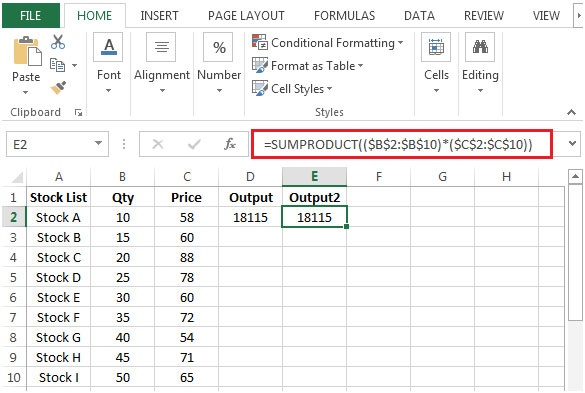Terms and Conditions of use

The applications/code on this site are distributed as is and without warranties or liability. In no event shall the owner of the copyrights, or the authors of the applications/code be liable for any loss of profit, any problems or any damage resulting from the use or evaluation of the applications/code.